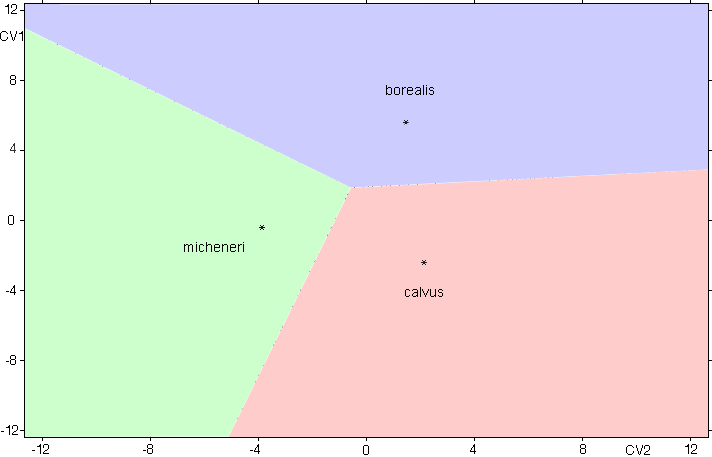*Group centroid indicated by an asterisk

Discrimination Among Three Forms of Mites of the Genus Chaetodactylus Associated with Bees of the Genus Osmia, Subgenus Cephalosmia
*All regulated by Code names used here are not available names. They will be available when the paper describing them will be published.

To classify an unknown specimen input raw measurements and, if necessary, scale coefficients (if measurements are in different scale)
and press the button "Classify Specimen" below

You can paste measurements from any spreadsheet application into the textbox below. Data must be arranged in a single row.

 Calculations involve the following formula: CV = b1*x1 + b2*x2 + ... + bn*xn + c b1,b2,...bn Coefficients for CV1-CVn x1,x2,...xn Darroch and Mosimann shape variables (measurement divided by the geometric mean of all variables of the given specimen) c Constant

Data:
Measurements used to develop and verify this classification model can be downloaded as a MS Excel file here
To interpret BMOC numbers, unique identifiers of mite specimens, visit our database